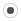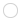## Generic code for sum between sparse and dense expression.svalorzen
Registered MemberPosts
3
Karma
0

### Generic code for sum between sparse and dense expression.

Tue Jan 26, 2016 1:25 am
I am writing a bunch of code in a library which I'd like to support both Sparse and Dense Eigen matrices, without the need to write two separate implementations of the code. Up until now I succeeded (I hope without sacrificing too much performance), but unfortunately I have just hit a bad case, and I'd like some help in how to proceed.

My problem results from this bug: http://eigen.tuxfamily.org/bz/show_bug.cgi?id=632, which basically says it is impossible to directly sum sparse and dense expressions together. It seems that even the 3.3 beta branch still has not fixed this.

My code looks as follows:

Code: Select all
`#include <Eigen/Core>#include <Eigen/SparseCore>#include <iostream>using Matrix2D = Eigen::Matrix<double, Eigen::Dynamic, Eigen::Dynamic, Eigen::RowMajor | Eigen::AutoAlign>;using SparseMatrix2D = Eigen::SparseMatrix<double, Eigen::RowMajor>;using Vector   = Eigen::Matrix<double, Eigen::Dynamic, 1>;int main() {    Matrix2D result(5,5);    // These may be sparse!    Matrix2D a(5,5);    Matrix2D b(5,5);    // Other possible input    // SparseMatrix2D a(5,5)    // SparseMatrix2D b(5,5)    a.fill(5.0);    b.fill(4.0);    // This is only dense    Vector v(5);    v.fill(1.0);    double someScalar = 4.0;    // !!! The important bit !!! This is actually in a template function which depends on the type of a and b    // Note that all indeces are zero for convenience in the example, they could be any number.    result(0,0) = b.row(0).dot(v.transpose() * someScalar + a.row(0));    // I use the result to do other stuff later.    std::cout << result(0,0) << "\n";    return 0;}`

My question is: would it be possible to rewrite the important bit line with a syntax valid when both a and b may be Sparse Matrices?

For example, one thing I found out was that I could do v.transpose().sparseView() to make it work if a and b are sparse, but that solution makes it not work when they are dense. Is there some way to write it once which does not have too much overhead in both cases?ggael
ModeratorPosts
3447
Karma
19
OS### Re: Generic code for sum between sparse and dense expression

Wed Jan 27, 2016 5:19 pm
You can do:

result(0,0) = someScalar * b.row(0).dot(v) + b.row(0).dot(a.row(0));

For 'a' dense, the complexity is the same because you factor out the scalar multiple, and for 'a' sparse, the complexity is even reduced. The downside is more memory reads to 'b' entries. Another solution would be to update v inplace (or to another vector):

v *= someScalar;
v += a.row(0).transpose();
b.row(0).dot(v);

This how Eigen would implement dense + sparse if supported because performing the operation on the fly would not allow to exploit the sparcity of 'a'. The last solution is to see 'v' as a sparse vector:

[...] v.sparseView(-1) + a

and does that only if 'a' is sparse, but my guess is that would be slowest solution.

## Who is online

Registered users: Bing [Bot], daret, Google [Bot], Will_S.